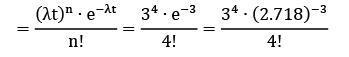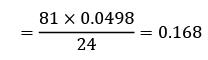Courses

# Test: Queueing Theory (Level - 2)

## 10 Questions MCQ Test Manufacturing and Industrial Engineering | Test: Queueing Theory (Level - 2)

Description
This mock test of Test: Queueing Theory (Level - 2) for Mechanical Engineering helps you for every Mechanical Engineering entrance exam. This contains 10 Multiple Choice Questions for Mechanical Engineering Test: Queueing Theory (Level - 2) (mcq) to study with solutions a complete question bank. The solved questions answers in this Test: Queueing Theory (Level - 2) quiz give you a good mix of easy questions and tough questions. Mechanical Engineering students definitely take this Test: Queueing Theory (Level - 2) exercise for a better result in the exam. You can find other Test: Queueing Theory (Level - 2) extra questions, long questions & short questions for Mechanical Engineering on EduRev as well by searching above.
*Answer can only contain numeric values
QUESTION: 1

### A person repairing radios finds that the time spent on the radio sets has exponential distribution with mean 20 minutes. If the radios are repaired in the order in which they come in and their arrival is approximately poisson with an average rate 15 for 8 hour day. What is the repairmen’s expected idle time each day?(A)2.8(B)3.2.

Solution: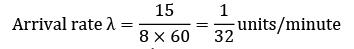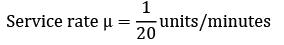No. of hours for which the repairman remains busy in an 8-hour day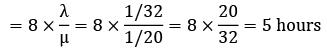∴ Time for which repairman remains idle

= 8 − 5

= 3 hours

QUESTION: 2

### Consider a single server queueing model where the mean arrival rate is 4 per 8 hour shift and the arrivals follow poisson distribution. The mean service time is 60 minutes and it follows exponential distribution. The idle time of the server is

Solution: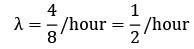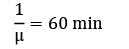μ = 1/hour

Idle time at the server = (1 − ρ) × 8 hours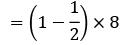= 4 hours

*Answer can only contain numeric values
QUESTION: 3

### In a small clinic only one case is possible to handle at a time, patients arrive on an average of 3 per hour. The mean service time should be less than _________ minutes per patient so that the average time in the system is less than 15 minutes.

Solution: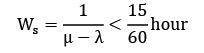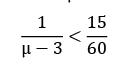μ < 7="" />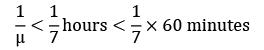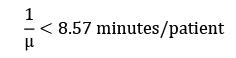*Answer can only contain numeric values
QUESTION: 4

A repair shop is manned with a single worker. Customers arrive at a rate of 20 per hour. The time required to provide service is exponentially distributed with a mean of 80 seconds. If the productivity loss for an hour is Rs. 20,000 due to breakdown, what could be the expected loss?

Solution:

Given,

Arrival rate = Rs. 20/hour = λ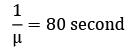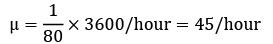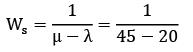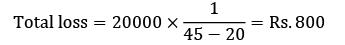*Answer can only contain numeric values
QUESTION: 5

A typist at an office of a company receives on an average 20 letters per day for typing. The typist works 8 hours a day and it takes on the average 20 minutes to type a letter. The cost of a letter waiting to be mailed is 80 paise per hour and the cost of the equipment plus salary of the typist is Rs. 45 per day. What will be the total daily cost of waiting letters to be mailed?

Solution:

λ = 20 /day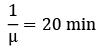Working hours = 8 hrs.

Cost of letter waiting to be mailed

= 80 paise/hr

= 0.8 Rs/hr.

Cost of equipment + Salary = Rs. 45/day

Total daily cost of waiting letters

= LS × cost/letter/hr × 8 +(cost of equipment + Salary)

= 5 × 0.8 × 8 + 45 = 32 + 45 = Rs. 77

*Answer can only contain numeric values
QUESTION: 6

Arrival rate of telephonic calls at a telephone booth are according to poisson distribution, with an average time of 9 minutes between two consecutive arrivals. The length of telephone call is assumed to be exponentially distributed with mean 3 minutes.

What is the probability that an arrival will have to wait for more than 10 minutes before the phone is free?

Solution:

Given,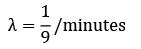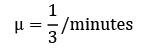Probability (waiting time ≥ 10)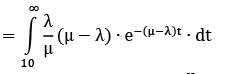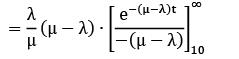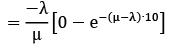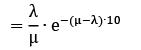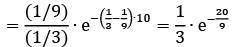= 0.033

*Answer can only contain numeric values
QUESTION: 7

The milk plant at a city distributes its products by trucks, loaded at the loading dock. It has its own fleet of trucks plus trucks of a private transport company. This transport company has complained that sometimes its trucks have to wait in line and thus the company loses money paid for a truck and driver that is only waiting. The company has asked the milk plant management either to go in for a second loading dock or discount prices equivalent to the waiting time. !rrival rate = 3/hour !verage service rate = 4/hour The transport company has provided 40% of the total number of trucks. The expected waiting time of company trucks per day before docking is __________

Solution:

Total expected waiting time of company trucks per day

= trucks/day × % of company trucks × expected waiting time per truck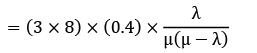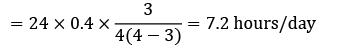*Answer can only contain numeric values
QUESTION: 8

In a large maintenance department, fitters draw parts from the parts store which is at present staffed by one store man. The maintenance foreman is concerned about the time spent by fitters getting parts and wants to know if the employment of a store labourer to assist the store man would be worthwhile. It was found that 1. fitters cost Rs.2.50/hour 2. store man costs Rs.2/hour and can deal on the average with 10 fitter per hour 3. a labourer could be employed at Rs.1.75/hour and would increase the service capacity of the store to 12/hour 4. on an average 8 fitters visit the store each hour What would be the net savings or loss if an extra labourer is employed?

Solution:

Without labourer: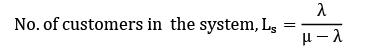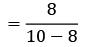= 4

Cost/hour = 4 × 2.50 = Rs. 10

With labourer: λ = 8/hour; μ = 12/hour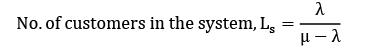`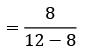= 2

Cost/hour = cost of fitters/hour + cost of labourer hour

= 2 × 2.50 + 1.75

= Rs. 6.75

Net savings = 10 − 6.75 = Rs 3.25

*Answer can only contain numeric values
QUESTION: 9

On an average 96 patients per 24-hours day require the service of an emergency clinic. Also on the average a patient requires 10 minute of active attention. Assume that the facility can handle only one emergency at a time suppose that it costs the clinic Rs.100 per patient treated to obtain an average servicing time of 10 minute, and that each minute of decrease in this average time would cost the clinic Rs.10 per patient treated. How much would have to be budgeted by the clinic to decrease the average size of the queue from

1

1

3

patients to 1/2 patients.

(A)123

(B)127

Solution: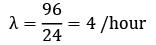μ = 1/10 × 60 = 6/hour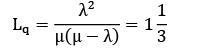This number is to be reduced from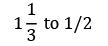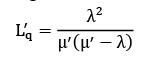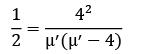μ′ = 8, −4

μ′ = 8

Average time required by each patient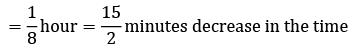required to attend a patient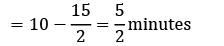∴ budget required for each patient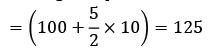*Answer can only contain numeric values
QUESTION: 10

On an average, 6 customers reach a telephonic booth every hour to make calls. Determine the probability that exactly 4 customers will reach in 30 min period, assuming that arrivals follow poisson distribution.

Solution:

λ = 6/hour

t = 30 min = 0.5 hours

n = 4

λt = 6 × 0.5 = 3 customers

Probability of 4 customers arriving in 0.5 hour